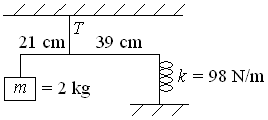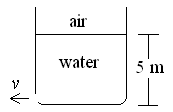First Name: ___________________ Last Name: ____________________ Section: _________

December 3, 1999 Physics 207

Exam 3

Print your name and section clearly on all five pages. (If you do not know your section number, write your TAs name.) Show all work in the space immediately below each problem. Your final answer must be placed in the box provided. Problems will be graded on reasoning and intermediate steps as well as on the final answer. Be sure to include units wherever necessary, and the direction of vectors. Each problem is worth 25 points. In doing the problems, try to be neat. Check your answers to see that they have the correct dimensions (units) and are the right order of magnitudes. You are allowed one 8½ x 11" sheet of notes and no other references. The exam lasts exactly 50 minutes.

(Do not write below)

SCORE:

Problem 1: __________

Problem 2: __________

Problem 3: __________

Problem 4: __________

TOTAL: ___________

First Name: ___________________ Last Name: ____________________ Section: _________

1. A horizontal massless rod, 60 cm long, is suspended 21 cm from one end by a wire as shown. A 2-kg mass hangs from the short end of the rod, and the long end is attached to a fixed support by a vertical spring with a spring constant of 98 N/m.a. What is the tension T in the wire? (15 pts.)

b. What is the frequency (in Hertz) of small-amplitude vertical oscillations of the mass m about its equilibrium position? (10 pts.)

First Name: ___________________ Last Name: ____________________ Section: _________

2. A spacecraft orbits the moon just above its surface. The radius of the moon is 1740 km, and its gravitational acceleration is one sixth that of Earth.

a. What is the speed of the spacecraft? (9 pts.)

b. What is the period of the spacecraft? (8 pts.)

c. What is the escape velocity from the moon? (8 pts.)

First Name: ___________________ Last Name: ____________________ Section: _________

3. A tank is filled with water to a depth of 5 m. The density of water is 1000 kg/m3 and the pressure of the atmosphere is 105 N/m2.a. What is the pressure at the bottom of the tank? (8 pts.)

b. With what velocity does water exit a small hole in the side of the tank at the bottom? (9 pts.)

c. If a layer of oil with a density of 700 kg/m3 is added to the water, bringing the level to 7 m, what is the pressure at the bottom of the tank? (8 pts.)

First Name: ___________________ Last Name: ____________________ Section: _________

4. A professor is lecturing in a monotone at a frequency of 200 Hz. The speed of sound in air is 343 m/s.

a. What is the wavelength of the sound? (5 pts.)

b. At what minimum speed and in what direction must the students move in order to Doppler shift his voice to a frequency too low to be heard? (10 pts.)

c. What is the closest distance between the professor and the blackboard for which the reflected wave interferes constructively with the direct sound from his mouth? (10 pts.)